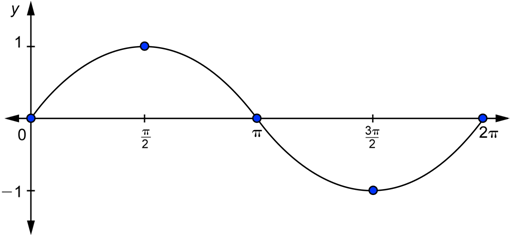• Astrophysics• Electricity• Electromagnetism• Energy• Fields• Force• Mechanics• Momentum• Nuclear Physics• Quantities & Units• States of Matter• Waves• Key ExperimentsWhen two waves overlap, they create a new wave with a different shape and size. This is called interference. Interference happens when two waves combine, and the result is a wave with a different amplitude and mathematical representation. If you're interested in how waves work, it's important to understand interference. It's a key concept in many different fields, from physics to engineering.

## Types of interference

Waves are characterized by three main attributes: amplitude, frequency, and phase. Amplitude is how big the wave is, frequency is how often the wave oscillates in a given time (usually a), phase is where the wave is in relation to a reference point.

To better understand these concepts, let's look at an example using the sinusoidal functions sin(x) and sin(x + π/2). These two waves have the same amplitude and frequency, but different phases. This means that they have the same shape, but are shifted in space. To see this visually, take look at graphs below.

The phase of a wave is important because it affects how waves overlap. In the graph above, the two waves have the same amplitude and frequency, but different phases. This causes the black wave to shift backwards, which we can see in the graph.

To understand phase differences, we use a reference point. In this case, the reference point is zero. The phase angle of the red wave sin(x) is zero, which means its starting point is the same as the reference point. For the black wave, the phase difference is positive (+π2), which means its starting point is before the reference point. This is why it shifts backwards on the graph.

When the phase difference is negative, the starting point of the wave is after the reference point. We can see this on the graph as the wave being to the right of the reference point. Understanding phase is crucial when studying waves, as it can have a significant impact on how they behave and interact with each other.

### Constructive and destructive interference

If we add up two identical waves, the resulting amplitude doubles:

sin (x) + sin (x) = 2 sin (x)

While mathematically obvious, have a look at what it means visually, with two sinusoids one above the other, as in figure 2.

The points that have the same x coordinate also have the same amplitude. Applying the sum causes the wave to stretch. Where the amplitude was 1, it is now 2, and where it was -1, it is now -2. This is called constructive interference. An example from everyday life is two speakers playing the same track. The volume of the music perceived is maximum when the waves produced by the speakers are in phase, interfering constructively.

When the waves have different phases, the result of the superposition changes, especially if they are in phase opposition or counter-phase when every point is added to one of the opposite value, e.g., 1 + (-1), as in the graph below.Sin (x - π/2) (blue), sin (x + π/2) (red). Their sum is a straight line

The sum of these waves is zero due to destructive interference. Notice how, in this case, the phase causes the same result as the negative sign before the function.

sin (x - π / 2) + sin (x + π / 2) = 0

sin (x - π / 2) - sin (x - π / 2) = 0

We defined the two ends of the line. In between, there are all the combinations of the two waves. The phase of the resulting wave is shifted to somewhere between the phases of the interfering waves, depending on their amplitudes, and the value of its amplitude will be between zero and twice the amplitude of the interfering waves.

### Interference patterns

Interference is a phenomenon that occurs when two waves are superimposed or overlap with each other. This can happen in one dimension, like with sound waves, or in two or more dimensions, like with waves on the surface of a lake. When two stones are thrown into a lake from slightly different spots, a bi-dimensional wave forms on the surface of the water. This creates an interference pattern, where the surface of the water becomes corrugated but still exhibits a regularity.

In the case of bi-dimensional waves, the position of the source (where the stones are thrown from) can also affect the pattern. The waves are much more corrugated near the sources and almost unaffected moving away from them.

Interference can be constructive or destructive, but usually, it's a combination of both. Interference patterns can occur in any case where waves propagate along one or more dimensions. It's important to understand the phenomenon of interference when studying waves, as it can have a significant impact on how they behave and interact with each other.

## Interference

What is destructive interference?

Destructive interference is the kind of interference that causes two waves to subtract each other.

What is the interference phenomenon?

Interference is the phenomenon that occurs when two waves collide with each other.

What is interference and what kinds of interference exist?

Interference is when two waves collide with each other. There are two types of interference: constructive and destructive interference.

What happens in interference?

Two waves collide, creating a wave that is the sum of the two.14-day free trial. Cancel anytime.Join 10,000+ learners worldwide.The first 14 days are on us96% of learners report x2 faster learningFree hands-on onboarding & supportCancel Anytime